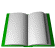## Dilbert's "Salary Theorem"

Dilbert's "Salary Theorem" states that, "geologists and engineers can never earn as much money as business executives, company lawyers, and sales people." This basic concept is easily proved by a mathematical equation based on the basic assumptions that "Knowledge is Power" and "Time is Money".

 As every engineer knows: Power = Work / Time Therefore if as assumed: Knowledge = Power And: Time = Money It follows that Knowledge = Work / Money

By Solving this equation for Money, we get:

Money = Work / Knowledge

Therefore, as Knowledge approaches zero, Money approaches infinity, regardless of the amount of Work done.

Conclusion: The less you know, the more you make.2/23/99